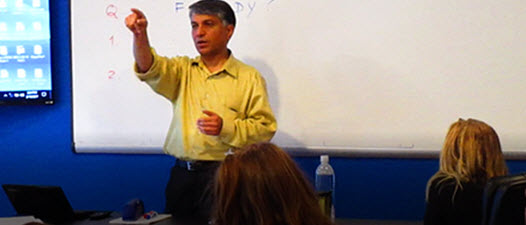GRE® Prep Classes
512.587.8694

# GRE Math Foundations

The 6-hour Math Foundations class covers fundamental arithmetic, algebra, and geometry to prepare you for the more advanced GRE math topics. This class is included in GRE Plus and GRE Premium for MBA Applicants courses.Math Foundations is included with GRE Plus and GRE Premium course packages.

#### Do You Need GRE Math Foundations?

Take the 10-minute self-assessment quiz below. You may use a pencil and paper. Because this is basic math, we recommend that you not use a calculator, so that you can truly assess your retained knowledge.

If you did not answer at least 6 questions correctly, you should consider our GRE Plus Course.

#### Basic Math Assessment Test

(0.5)3 =
Oops! Something went wrong while submitting the form.
Correct!
Sorry, the correct answer is 0.125.10% of 150 =
Oops! Something went wrong while submitting the form.
Correct!
Sorry, the correct answer is 15.The square root of 0.4 is approximately
Oops! Something went wrong while submitting the form.
Correct!
Sorry, the correct answer is 0.6.For any real number X, the expression X3 + X3 =
Oops! Something went wrong while submitting the form.
Correct!
Sorry, the correct answer is 2x3.If X/5 is subtracted from X/3, the answer is
Oops! Something went wrong while submitting the form.
Correct!
Sorry, the correct answer is 2X/15.If M monkeys share a total of B bananas equally, how many bananas would each monkey get?
Oops! Something went wrong while submitting the form.
Correct!
Sorry, the correct answer is B/M.In triangle ABC, if angle A is 120 degrees, then angle B cannot be
Oops! Something went wrong while submitting the form.
Correct!
Sorry, the correct answer is 70 degrees.What is the maximum possible straight line distance between any two points on a circle of radius 1?
Oops! Something went wrong while submitting the form.
Correct!
Sorry, the correct answer is 2.What is the area of a square whose perimeter is 4?
Oops! Something went wrong while submitting the form.
Correct!
Sorry, the correct answer is 1.If u > t, r > q, s > t, and t > r, which of the following must be true?

I. u > s
II. s > q
III. u > r
Oops! Something went wrong while submitting the form.
Correct! And the quiz is complete.
Sorry, the answer is II and III. And the quiz is complete.Here is the answer sheet, but remember that you won't be allowed to bring notes into the GRE exam!
Q1. (0.5)3 =
A1. 0.125
Q2. 10% of 150 =
A2. 15
Q3. The square root of 0.4 is approximately
A3. 0.6
Q4. For any real number X, the expression X3 + X3 =
A4. 2X3
Q5. If X/5 is subtracted from X/3, the answer is
A5. 2X/15
Q6. If M monkeys share a total of B bananas equally, how many bananas would each monkey get?
A6. B/M
Q7. In triangle ABC, if angle A is 120 degrees, then angle B cannot be
A7. 70 degrees
Q8. What is the maximum possible straight line distance between any two points on a circle of radius 1?
A8. 2
Q9. What is the area of a square whose perimeter is 4?
A9. 1
Q10. If u > t, r > q, s > t, and t > r, which of the following must be true?
I. u > s
II. s > q
III. u > r
A10. II and III
Oops! Something went wrong while submitting the form.
You've Got Options

# GRE Plus

45+ Hours of GRE Prep
with Extra Math Support